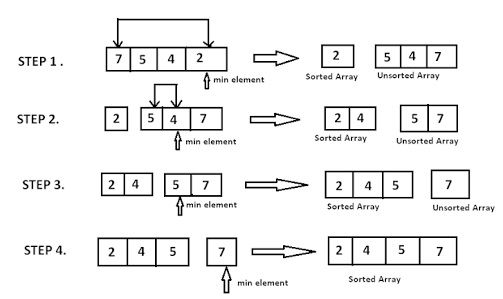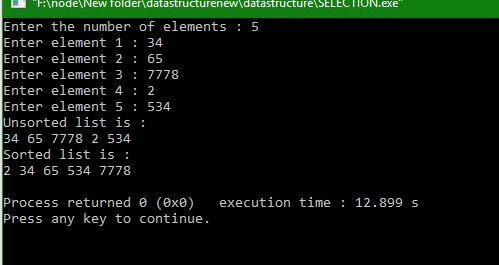# How to use Selection Sort in Data Structure?

In this article, we are going to learn what selection is sorting and how to implement Selection Sort?
Submitted by Manu Jemini, on January 24, 2018Image source: https://encrypted-tbn2.gstatic.com/images?q=tbn:ANd9GcQtJavqWTdGtHc6VbKnezustEX5Ea0ZHc8Zalr0vzq4ZBMmGqN-jbLslG8HYA

The Example above in the image is very decent to understand the selection sort algorithm.

In Selection Sort we make a pass in the array and select the smallest element in the array. In the end, we swap the selected element with the 0th index and update the index counter.

This will again repeat for the array but we will leave the 0th index because it is the smallest element of the array. So the smallest element in the array from the index 1 to n will be placed at the index 1.

This sorting is a type of linear sort and also slower than the Quick and merge sorts.

## C program to implement selection sort

```#include <stdio.h>
#define MAX 20

void selection(int arr[],int n)
{
/*Selection sort*/
int i,smallest,k,temp;
for(i = 0; i< n - 1 ; i++)
{
/*Find the smallest element*/
smallest = i;
for(k = i + 1; k < n ; k++)
{
if(arr[smallest] > arr[k])
smallest = k ;
}
if( i != smallest )
{
temp = arr [i];
arr[i] = arr[smallest];
arr[smallest] = temp ;
}
}/*End of for*/
}

//main code
int main()
{

int arr[MAX], i,n;
printf("Enter the number of elements : ");
scanf("%d",&n);

for (i = 0; i < n; i++)
{
printf("Enter element %d : ",i+1);
scanf("%d", &arr[i]);
}

printf("Unsorted list is : \n");
for (i = 0; i < n; i++)
printf("%d ", arr[i]);
printf("\n");

selection(arr,n);

printf("Sorted list is : \n");
for (i = 0; i < n; i++)
printf("%d ", arr[i]);

printf("\n");

return 0;
}/*End of main()*/
```

Output